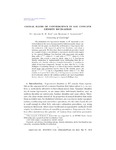## GLOBAL RATES OF CONVERGENCE IN LOG-CONCAVE DENSITY ESTIMATION

##### Repository DOIArticle
##### Authors
Kim, Arlene KH
Samworth, Richard J
##### Abstract

The estimation of a log-concave density on Rd represents a central problem in the area of nonparametric inference under shape constraints. In this paper, we study the performance of log-concave density estimators with respect to global loss functions, and adopt a minimax approach. We first show that no statistical procedure based on a sample of size n can estimate a log-concave density with respect to the squared Hellinger loss function with supremum risk smaller than order n−4/5, when d=1, and order n−2/(d+1) when d≥2. In particular, this reveals a sense in which, when d≥3, log-concave density estimation is fundamentally more challenging than the estimation of a density with two bounded derivatives (a problem to which it has been compared). Second, we show that for d≤3, the Hellinger ε-bracketing entropy of a class of log-concave densities with small mean and covariance matrix close to the identity grows like max{ε−d/2,ε−(d−1)} (up to a logarithmic factor when d=2). This enables us to prove that when d≤3 the log-concave maximum likelihood estimator achieves the minimax optimal rate (up to logarithmic factors when d=2,3) with respect to squared Hellinger loss.

##### Keywords
Bracketing entropy, density estimation, global loss function, log-concavity, maximum likelihood estimation
##### Journal Title
ANNALS OF STATISTICS
0090-5364
44
Project Euclid